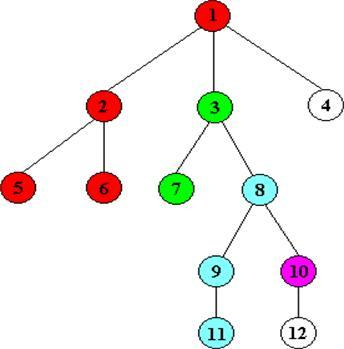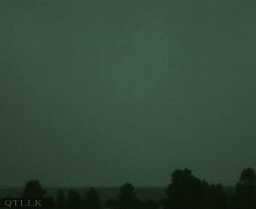# dfs和bfs

`https://www.cnblogs.com/wzl19981116/p/9397203.html`

# 1.dfs(深度优先搜索)是两个搜索中先理解并使用的，其实就是暴力把所有的路径都搜索出来，它运用了回溯，保存这次的位置，深入搜索，都搜索完了便回溯回来，搜下一个位置，直到把所有最深位置都搜一遍，要注意的一点是，搜索的时候有记录走过的位置，标记完后可能要改回来；### 理解这种方法比较简单，难的是要怎么用

``void dfs(int deep) { int x=deep/n,y=deep%n; if(符合某种要求||已经不能在搜了) { 做一些操作； return ; } if(符合某种条件且有地方可以继续搜索的)//这里可能会有多种条件，可能要循环什么的 { a[x][y]='x';//可能要改变条件，这个是瞎写的 dfs(deep+1,sum+1);//搜索下一层 a[x][y]='.';//可能要改回条件，有些可能不用改比如搜地图上有多少块连续的东西 } }``

# 2.bfs(宽度/广度优先搜索)，这个一直理解了思想，不会用，后面才会的，思想，从某点开始，走四面可以走的路，然后在从这些路，在找可以走的路，直到最先找到符合条件的，这个运用需要用到队列(queue)，需要稍微掌握这个才能用bfs。``````int visit[N][N]//用来记录走过的位置
int dir={0,-1,0,1,-1,0,1,0}; struct node { int x,y,bits;//一般是点，还有步数，也可以存其他的 }; queue<node>v; void bfs1(node p) { node t,tt; v.push(p); while(!v.empty()) { t=v.front();//取出最前面的 v.pop();//删除 if(找到符合条件的) { 做记录； while(!v.empty()) v.pop();//如果后面还需要用，随手清空队列 return; } visit[t.x][t.y]=1;//走过的进行标记，以免重复 rep(i,0,4)//做多次查找 { tt=t; tt.x+=dir[i];tt.y+=dir[i];//这里的例子是向上下左右查找的 if(如果这个位置符合条件) { tt.bits++;//步数加一 v.push(tt); //把它推入队列，在后面的时候就可以用了 } } } }``````

# 1.dfs(深度优先搜索)是两个搜索中先理解并使用的，其实就是暴力把所有的路径都搜索出来，它运用了回溯，保存这次的位置，深入搜索，都搜索完了便回溯回来，搜下一个位置，直到把所有最深位置都搜一遍，要注意的一点是，搜索的时候有记录走过的位置，标记完后可能要改回来；### 理解这种方法比较简单，难的是要怎么用

``void dfs(int deep) { int x=deep/n,y=deep%n; if(符合某种要求||已经不能在搜了) { 做一些操作； return ; } if(符合某种条件且有地方可以继续搜索的)//这里可能会有多种条件，可能要循环什么的 { a[x][y]='x';//可能要改变条件，这个是瞎写的 dfs(deep+1,sum+1);//搜索下一层 a[x][y]='.';//可能要改回条件，有些可能不用改比如搜地图上有多少块连续的东西 } }``

# 2.bfs(宽度/广度优先搜索)，这个一直理解了思想，不会用，后面才会的，思想，从某点开始，走四面可以走的路，然后在从这些路，在找可以走的路，直到最先找到符合条件的，这个运用需要用到队列(queue)，需要稍微掌握这个才能用bfs。``````int visit[N][N]//用来记录走过的位置
int dir={0,-1,0,1,-1,0,1,0}; struct node { int x,y,bits;//一般是点，还有步数，也可以存其他的 }; queue<node>v; void bfs1(node p) { node t,tt; v.push(p); while(!v.empty()) { t=v.front();//取出最前面的 v.pop();//删除 if(找到符合条件的) { 做记录； while(!v.empty()) v.pop();//如果后面还需要用，随手清空队列 return; } visit[t.x][t.y]=1;//走过的进行标记，以免重复 rep(i,0,4)//做多次查找 { tt=t; tt.x+=dir[i];tt.y+=dir[i];//这里的例子是向上下左右查找的 if(如果这个位置符合条件) { tt.bits++;//步数加一 v.push(tt); //把它推入队列，在后面的时候就可以用了 } } } }``````